# matlab神经网络预测建模,浅层神经网络时序预测和建模

targets = {1 2 3 4 5};

nnstart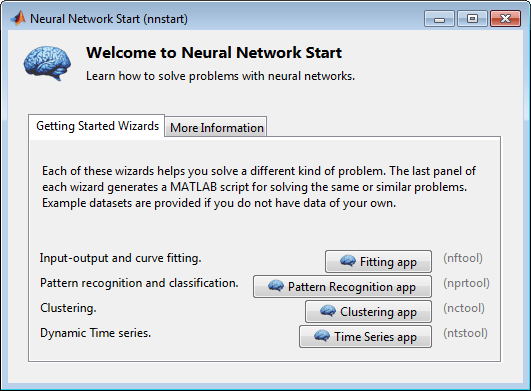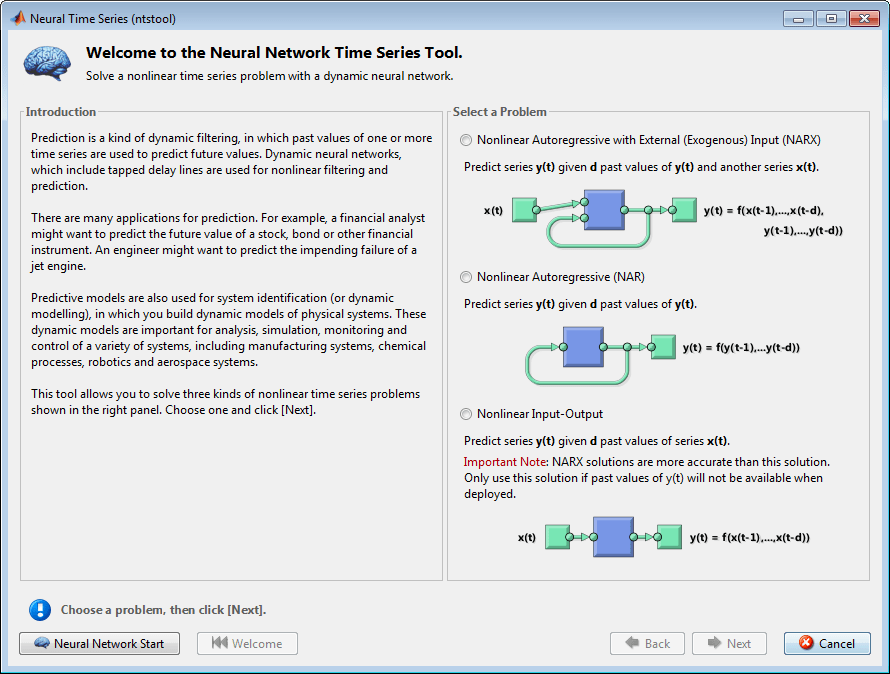y(t) = f(y(t – 1), ..., y(t – d), x(t – 1), ..., (t – d))

y(t) = f(y(t – 1), ..., y(t – d))

y(t) = f(x(t – 1), ..., x(t – d))

NARX 模型将提供比该输入-输出模型更好的预测，因为它使用包含在 y(t) 的先前值中的附加信息。但是，在某些应用中，y(t) 的先前值不可用。只有在这种情况下，才会使用输入-输出模型，而不是 NARX 模型。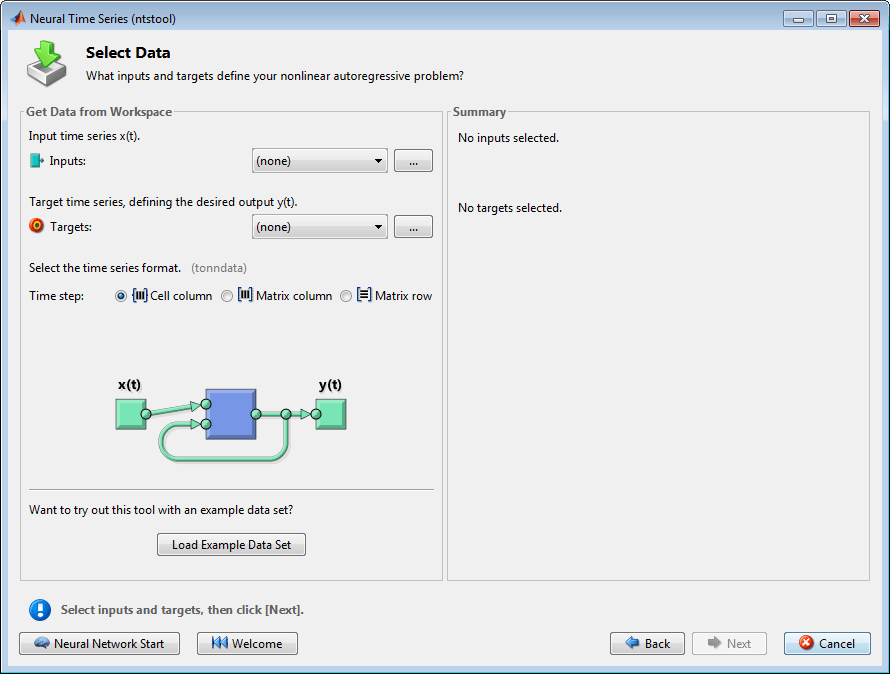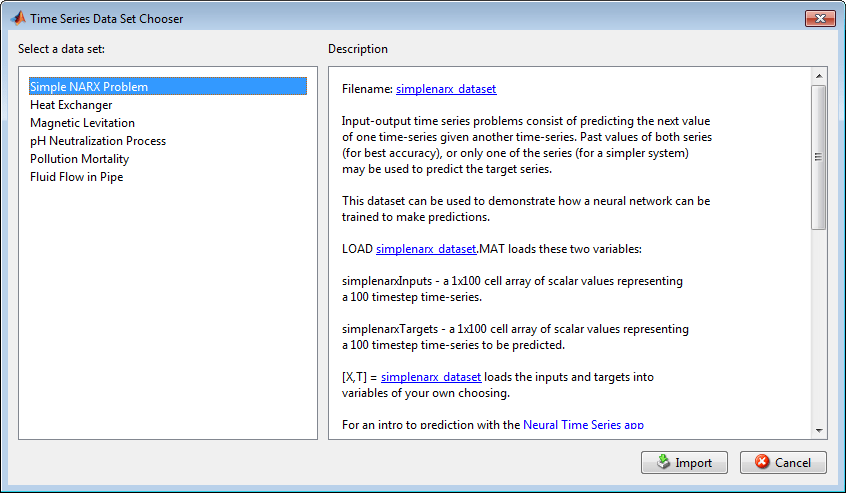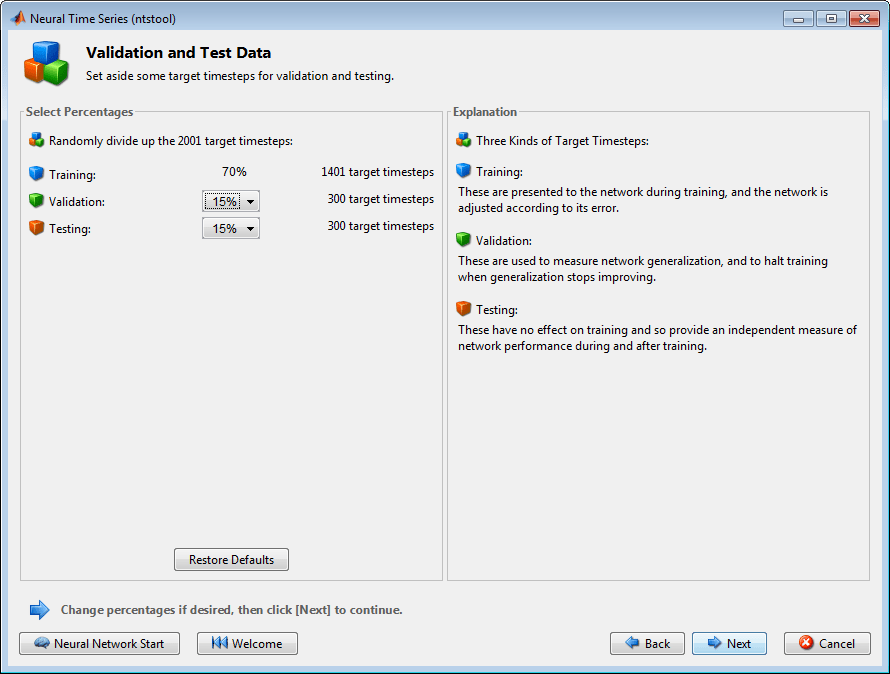70% 将用于训练。

15% 将用于验证网络是否正在泛化，并在过拟合前停止训练。

(有关数据划分流程的更多讨论，请参阅划分数据。)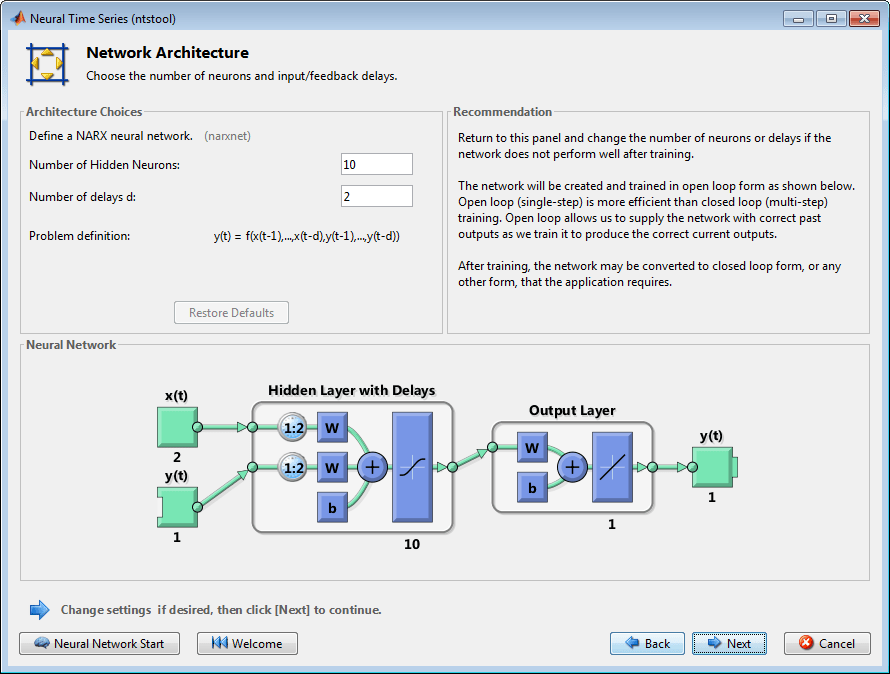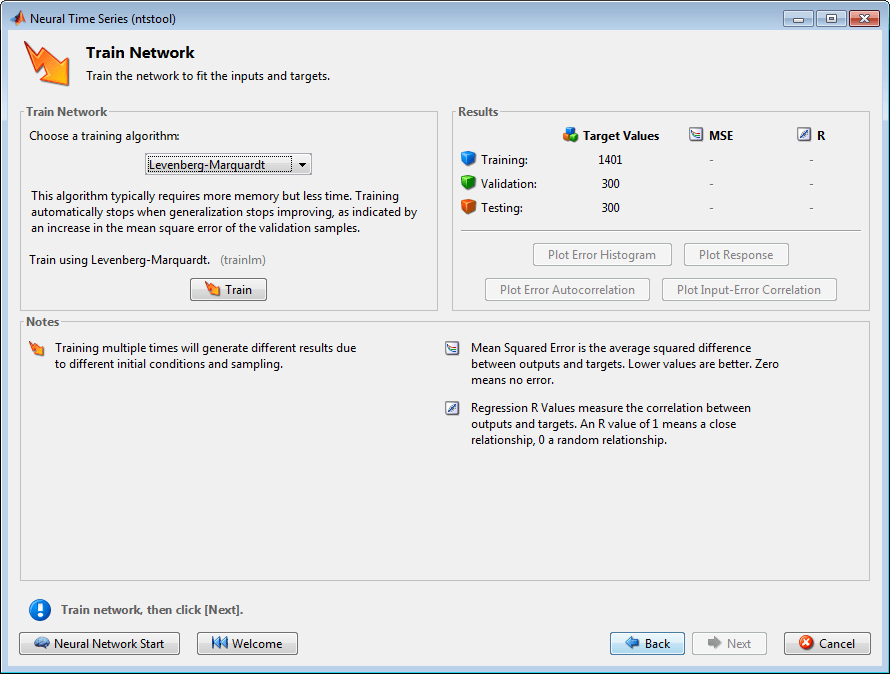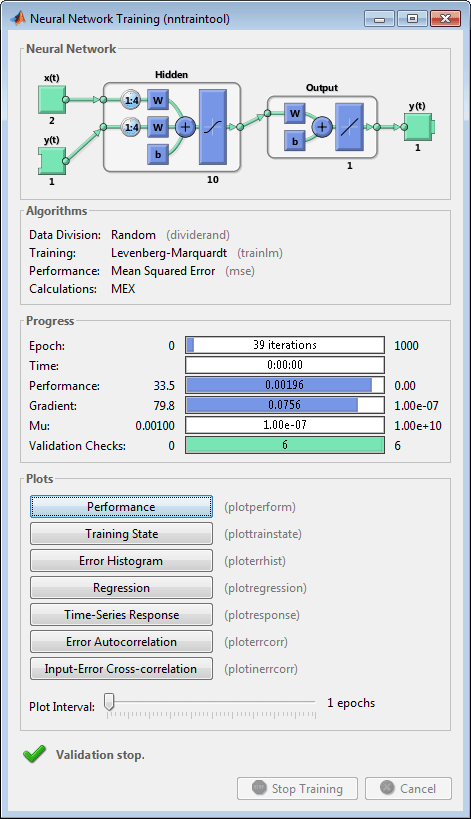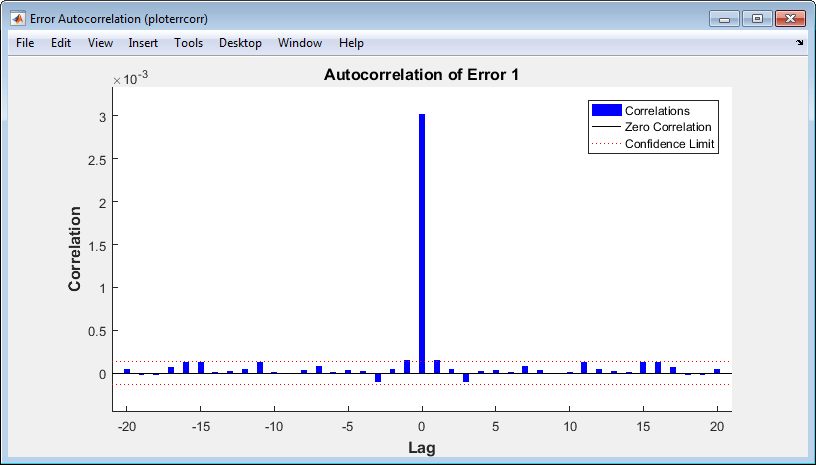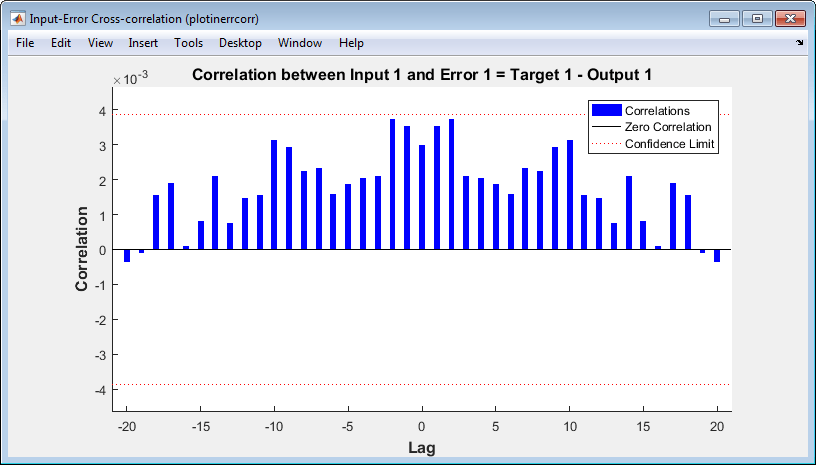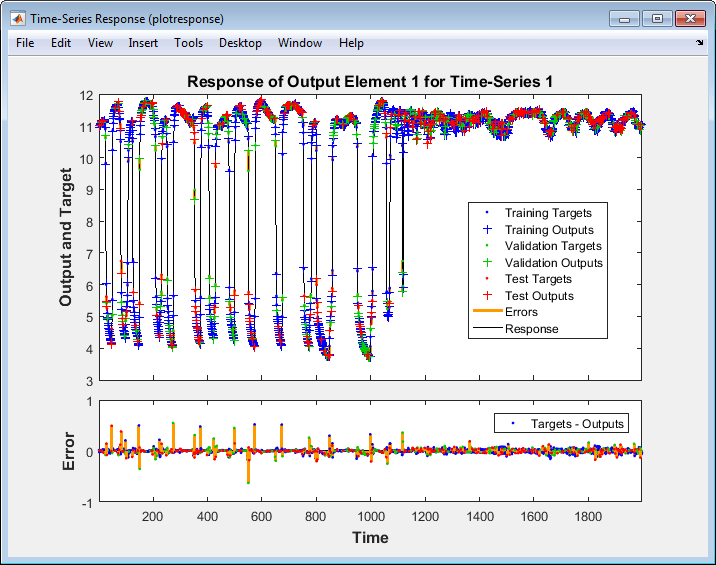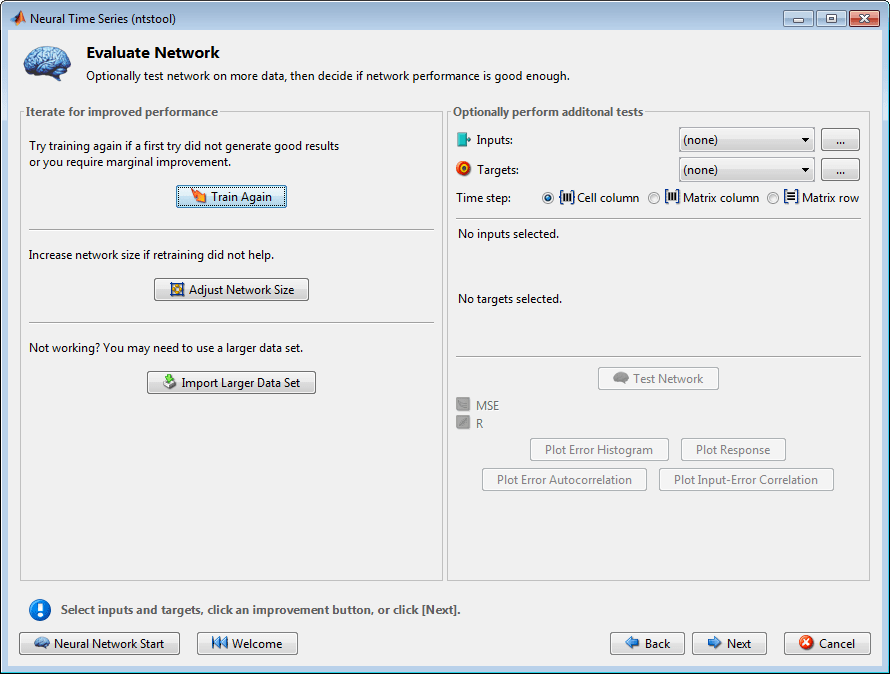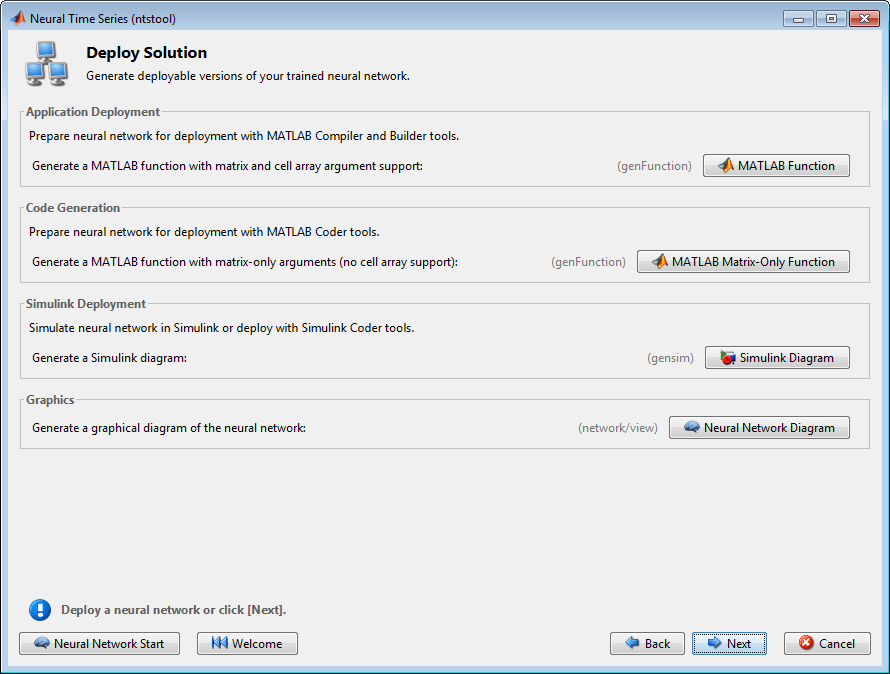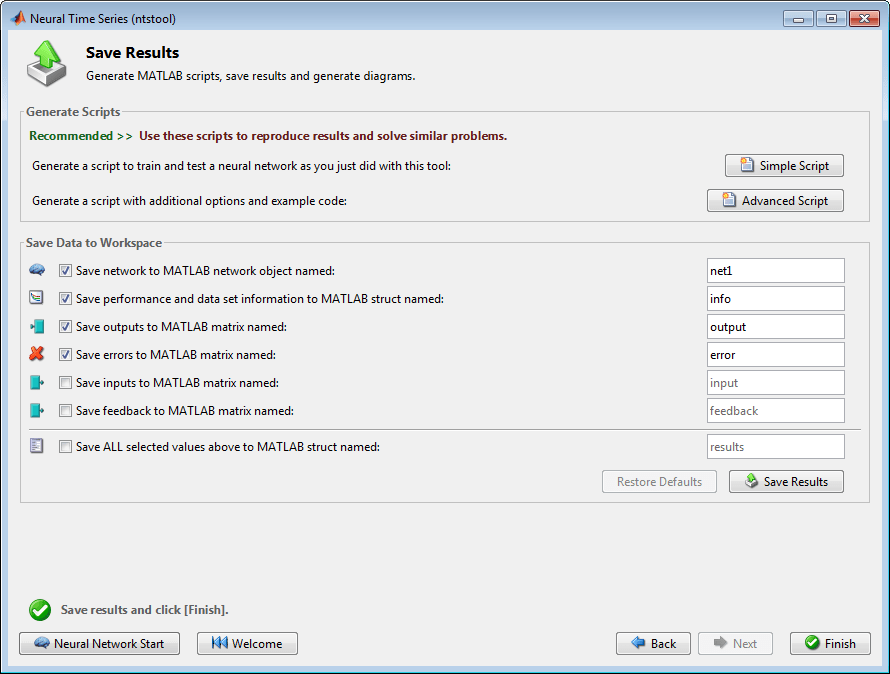% Solve an Autoregression Problem with External

% Input with a NARX Neural Network

% Script generated by NTSTOOL

%

% This script assumes the variables on the right of

% these equalities are defined:

%

% phInputs - input time series.

% phTargets - feedback time series.

inputSeries = phInputs;

targetSeries = phTargets;

% Create a Nonlinear Autoregressive Network with External Input

inputDelays = 1:4;

feedbackDelays = 1:4;

hiddenLayerSize = 10;

net = narxnet(inputDelays,feedbackDelays,hiddenLayerSize);

% Prepare the Data for Training and Simulation

% The function PREPARETS prepares time series data

% for a particular network, shifting time by the minimum

% amount to fill input states and layer states.

% Using PREPARETS allows you to keep your original

% time series data unchanged, while easily customizing it

% for networks with differing numbers of delays, with

% open loop or closed loop feedback modes.

[inputs,inputStates,layerStates,targets] = ...

preparets(net,inputSeries,{},targetSeries);

% Set up Division of Data for Training, Validation, Testing

net.divideParam.trainRatio = 70/100;

net.divideParam.valRatio = 15/100;

net.divideParam.testRatio = 15/100;

% Train the Network

[net,tr] = train(net,inputs,targets,inputStates,layerStates);

% Test the Network

outputs = net(inputs,inputStates,layerStates);

errors = gsubtract(targets,outputs);

performance = perform(net,targets,outputs)

% View the Network

view(net)

% Plots

% Uncomment these lines to enable various plots.

% figure, plotperform(tr)

% figure, plottrainstate(tr)

% figure, plotregression(targets,outputs)

% figure, plotresponse(targets,outputs)

% figure, ploterrcorr(errors)

% figure, plotinerrcorr(inputs,errors)

% Closed Loop Network

% Use this network to do multi-step prediction.

% The function CLOSELOOP replaces the feedback input with a direct

% connection from the output layer.

netc = closeloop(net);

netc.name = [net.name ' - Closed Loop'];

view(netc)

[xc,xic,aic,tc] = preparets(netc,inputSeries,{},targetSeries);

yc = netc(xc,xic,aic);

closedLoopPerformance = perform(netc,tc,yc)

% Early Prediction Network

% For some applications it helps to get the prediction a

% timestep early.

% The original network returns predicted y(t+1) at the same

% time it is given y(t+1).

% For some applications such as decision making, it would

% help to have predicted y(t+1) once y(t) is available, but

% before the actual y(t+1) occurs.

% The network can be made to return its output a timestep early

% by removing one delay so that its minimal tap delay is now

% 0 instead of 1. The new network returns the same outputs as

% the original network, but outputs are shifted left one timestep.

nets = removedelay(net);

nets.name = [net.name ' - Predict One Step Ahead'];

view(nets)

[xs,xis,ais,ts] = preparets(nets,inputSeries,{},targetSeries);

ys = nets(xs,xis,ais);

earlyPredictPerformance = perform(nets,ts,ys)

inputSeries = phInputs;

targetSeries = phTargets;

inputDelays = 1:4;

feedbackDelays = 1:4;

hiddenLayerSize = 10;

net = narxnet(inputDelays,feedbackDelays,hiddenLayerSize);

[inputs,inputStates,layerStates,targets] = ...

preparets(net,inputSeries,{},targetSeries);

net.divideParam.trainRatio = 70/100;

net.divideParam.valRatio = 15/100;

net.divideParam.testRatio = 15/100;

net.trainFcn = 'trainbr';

net.trainFcn = 'trainscg';

[net,tr] = train(net,inputs,targets,inputStates,layerStates);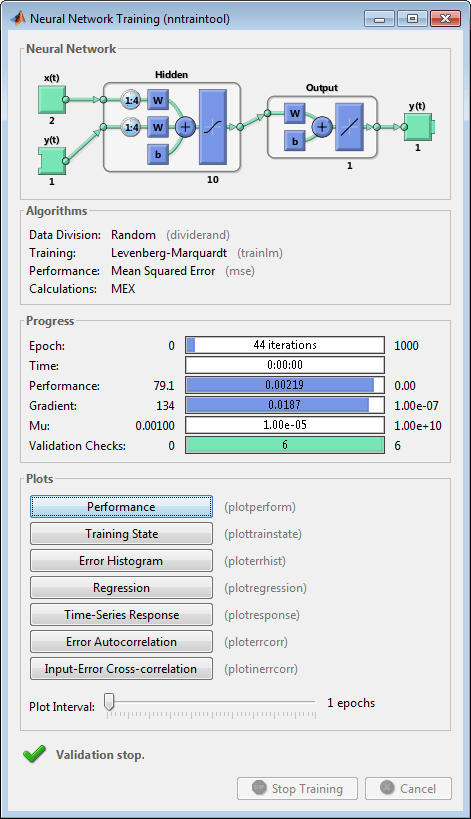outputs = net(inputs,inputStates,layerStates);

errors = gsubtract(targets,outputs);

performance = perform(net,targets,outputs)

performance =

0.0042

view(net)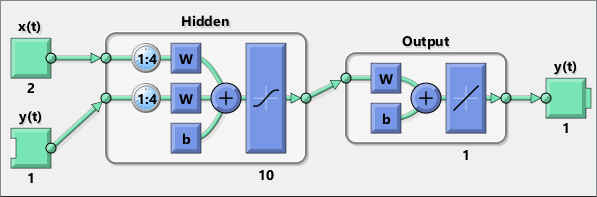figure, plotperform(tr)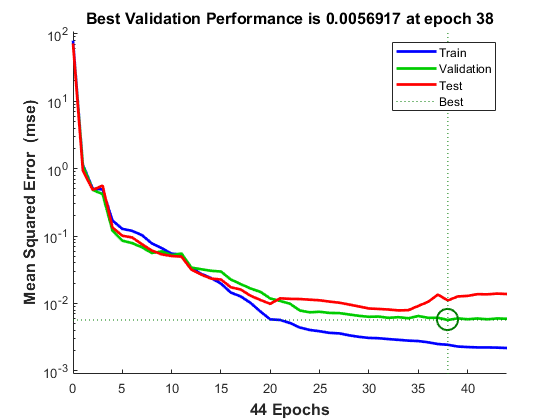netc = closeloop(net);

netc.name = [net.name ' - Closed Loop'];

view(netc)

[xc,xic,aic,tc] = preparets(netc,inputSeries,{},targetSeries);

yc = netc(xc,xic,aic);

perfc = perform(netc,tc,yc)

perfc =

2.8744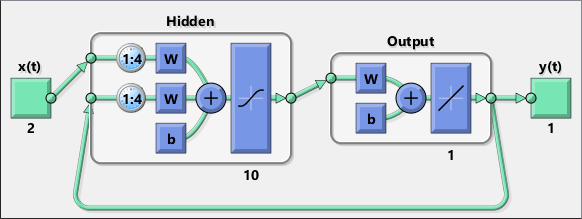nets = removedelay(net);

nets.name = [net.name ' - Predict One Step Ahead'];

view(nets)

[xs,xis,ais,ts] = preparets(nets,inputSeries,{},targetSeries);

ys = nets(xs,xis,ais);

earlyPredictPerformance = perform(nets,ts,ys)

earlyPredictPerformance =

0.0042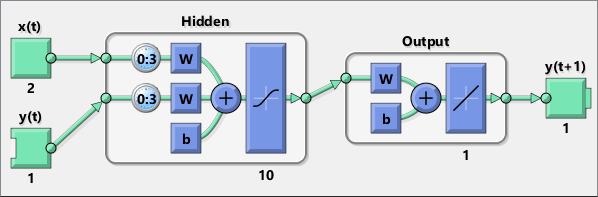07-289121
08-066672

01-071483
09-265889
11-2114万+
11-23816
02-053753
07-053659
03-16218
02-263万+
01-162619
07-039万+
07-031万+
12-025349
06-304075
06-011万+
03-041902
02-28432
03-162487
06-10951

### “相关推荐”对你有帮助么？

•非常没帮助
•没帮助
•一般
•有帮助
•非常有帮助点击重新获取扫码支付余额充值